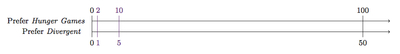# Hunger Games versus Divergent

Alignments to Content Standards: 6.RP.A 6.RP.A.3

The 150 students at Skokie School were asked if they prefer seeing the movie Hunger Games or Divergent. The data showed that 100 preferred Hunger Games and 50 preferred Divergent.

1. Look at the following statements and decide if each accurately reports the results of the survey and explain how you know.
1. At Skokie School, 1/3 of the students prefer Hunger Games.
2. Students prefer Hunger Games to Divergent in a ratio of 2 to 1.
3. The ratio of students who prefer Divergent to students who prefer Hunger Games is 1 to 2.
4. The number of students who prefer Hunger Games is 50 more than the number of students who prefer Divergent.
5. The number of students who prefer Hunger Games is two times the number of students who prefer Divergent
2. Compare statements (iv) and (v) above.  In what ways is the information given by these statements similar? In what ways is it different? Explain.

## IM Commentary

The goal of this task is to introduce ratio language and have students apply reasoning about ratios in a context.  In order to answer the questions, students need to understand the difference between a fraction and a ratio and need to analyze the part to whole and the part to part relationships in a ratio.

This is an engaging introductory lesson for a unit on ratio and proportional relationships.  Students can relate to the context and the numbers are "friendly" enough that students can focus on the concepts without getting bogged down in calculations.

This task was written as part of a collaborative project between Illustrative Mathematics, the Smarter Balanced Digital Library, the Teaching Channel, and Desmos.

## Solution

1. There are 150 students who took the survey so 1/3 of the students would be 50 students. But 100 of the students surveyed preferred Hunger Games to Divergent so this is not true. It is an easy mistake to make, however, because it is true that 1/3 more of the 150 students prefer Hunger Games compared to those who prefer Divergent.
2. The ratio of students who prefer Hunger Games to students who prefer Divergent is 100:50. This is equivalent to the ratio 2:1 as we can see by multiplying 2 and 1 by 50. This can also be shown in steps with a ratio table:

Students who prefer Hunger Games Students who prefer Divergent
100 50
10 5
2 1

The second line comes from the first by multiplying both entries by $\frac{1}{10}$ and then the third row is the second row multiplied by $\frac{1}{5}$.

3. The ratio of students who prefer Divergent to students who prefer Hunger Games is 50:100. This is equivalent to 1:2 as we can see by multiplying 1 and 2 by 50. This can be shown with the ratio table of part (b) or with a double number line as below:The given information is furthest to the right and then the equivalent ratios, calculated by multiplying by $\frac{1}{10}$ and then $\frac{1}{5}$, are to the left closer to 0.

4. Since 100 students prefer Hunger Games and 50 prefer Divergent and 100 = 50 + 50 it is true that 50 more students prefer Hunger Games.
5. Since 100 students prefer Hunger Games and 50 prefer Divergent and 100 = 2 $\times$ 50 it is true that the number of students who Hunger Games is twice the number who prefer Divergent.
1. The two statements give the same information if we know how many students took the survey. If we know that 150 students took the survey then both (d) and (e) tell us that 100 students preferred Hunger Games and 50 preferred Divergent. On the other hand, without this information only (e) tells us the ratio of students who preferred Hunger Games to Divergent